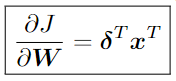Hi! After reading the material below, I have a question.

According to ch2 useful identities (5), (6) in the pdf above, the formula is defined as follows.

(5) Matrix times column vector with respect to the matrix

(6) Row vector time matrix with respect to the matrixI was wondering if this would actually be true, so I implemented it with pytorch

import random
import numpy as np
import torch

def set_seed(seed: int = 42):
"""Seed fixer (random, numpy, torch)
Args:
seed (:obj:int): The seed to set.
"""
random.seed(seed)
np.random.seed(seed)
torch.manual_seed(seed)
torch.cuda.manual_seed(seed)
torch.cuda.manual_seed_all(seed)  # if use multi-GPU
torch.backends.cudnn.deterministic = True
torch.backends.cudnn.benchmark = False

set_seed()

# (5) column vector case

y = W @ x

torch.isclose(
torch.ones_like(y) @ x.T, # delta * x^T
)

# (6) row vector case

y = f(x)

assert torch.isclose(
x.T @ torch.ones_like(y), # x^T * delta
# Since
nn.Linear in torch stores weights as transpose